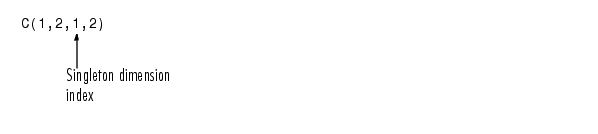ProgrammingCreating Multidimensional Arrays

You can use the same techniques to create multidimensional arrays that you use for two-dimensional matrices. In addition, MATLAB provides a special concatenation function that is useful for building multidimensional arrays.

This section discusses

Generating Arrays Using Indexing

One way to create a multidimensional array is to create a two-dimensional array and extend it. For example, begin with a simple two-dimensional array `A`.

• ```A = [5 7 8; 0 1 9; 4 3 6];
```

`A` is a 3-by-3 array, that is, its row dimension is 3 and its column dimension is 3. To add a third dimension to `A`,

• ```A(:,:,2) = [1 0 4; 3 5 6; 9 8 7]
```

MATLAB responds with

• ```A(:,:,1) =
5     7     8
0     1     9
4     3     6

A(:,:,2) =
1     0     4
3     5     6
9     8     7
```

You can continue to add rows, columns, or pages to the array using similar assignment statements.

Extending Multidimensional Arrays

To extend `A` in any dimension:

• Increment or add the appropriate subscript and assign the desired values.
• Assign the same number of elements to corresponding array dimensions. For numeric arrays, all rows must have the same number of elements, all pages must have the same number of rows and columns, and so on.

You can take advantage of the MATLAB scalar expansion capabilities, together with the colon operator, to fill an entire dimension with a single value:

• ```A(:,:,3) = 5;

A(:,:,3)
ans =
5     5     5
5     5     5
5     5     5
```

To turn `A` into a 3-by-3-by-3-by-2, four-dimensional array, enter

• ```A(:,:,1,2) = [1 2 3; 4 5 6; 7 8 9];
A(:,:,2,2) = [9 8 7; 6 5 4; 3 2 1];
A(:,:,3,2) = [1 0 1; 1 1 0; 0 1 1];
```

Note that after the first two assignments MATLAB pads `A` with zeros, as needed, to maintain the corresponding sizes of dimensions.

Generating Arrays Using MATLAB Functions

You can use MATLAB functions such as `randn`, `ones`, and `zeros` to generate multidimensional arrays in the same way you use them for two-dimensional arrays. Each argument you supply represents the size of the corresponding dimension in the resulting array. For example, to create a 4-by-3-by-2 array of normally distributed random numbers:

• ```B = randn(4,3,2)
```

To generate an array filled with a single constant value, use the `repmat` function. `repmat` replicates an array (in this case, a 1-by-1 array) through a vector of array dimensions.

• ```B = repmat(5, [3 4 2])

B(:,:,1) =
5     5     5     5
5     5     5     5
5     5     5     5

B(:,:,2) =
5     5     5     5
5     5     5     5
5     5     5     5
```

 Note    Any dimension of an array can have size zero, making it a form of empty array. For example, 10-by-0-by-20 is a valid size for a multidimensional array.

Building Multidimensional Arrays with the cat Function

The `cat` function is a simple way to build multidimensional arrays; it concatenates a list of arrays along a specified dimension:

• ```B = cat(`dim, A1, A2`...)
```

where `A1`, `A2`, and so on are the arrays to concatenate, and `dim` is the dimension along which to concatenate the arrays.

For example, to create a new array with `cat`:

• ```B = cat(3, [2 8; 0 5], [1 3; 7 9])

B(:,:,1) =
2     8
0     5

B(:,:,2) =
1     3
7     9
```

The `cat` function accepts any combination of existing and new data. In addition, you can nest calls to `cat`. The lines below, for example, create a four-dimensional array.

• ```A = cat(3, [9 2; 6 5], [7 1; 8 4])
B = cat(3, [3 5; 0 1], [5 6; 2 1])
D = cat(4, A, B, cat(3, [1 2; 3 4], [4 3;2 1]))
```

`cat` automatically adds subscripts of 1 between dimensions, if necessary. For example, to create a 2-by-2-by-1-by-2 array, enter

• ```C = cat(4, [1 2; 4 5], [7 8; 3 2])
```

In the previous case, `cat` inserts as many singleton dimensions as needed to create a four-dimensional array whose last dimension is not a singleton dimension. If the `dim` argument had been `5`, the previous statement would have produced a 2-by-2-by-1-by-1-by-2 array. This adds additional `1`s to indexing expressions for the array. To access the value `8` in the four-dimensional case, useMultidimensional Arrays Accessing Multidimensional Array Properties© 1994-2005 The MathWorks, Inc.